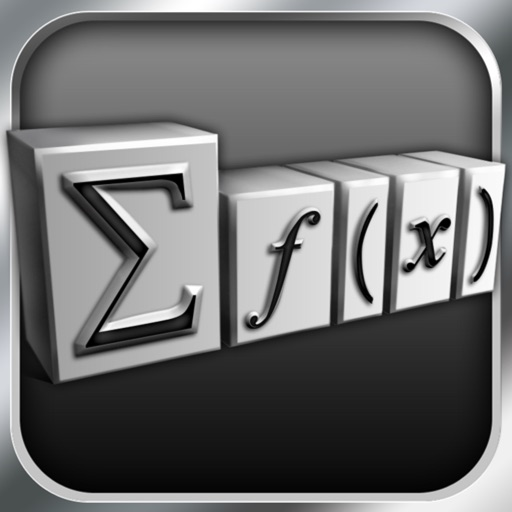## TeX Equation typesets math equations/formulas with built-in TeX engine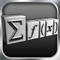# TeX Equation

by Chaoji Li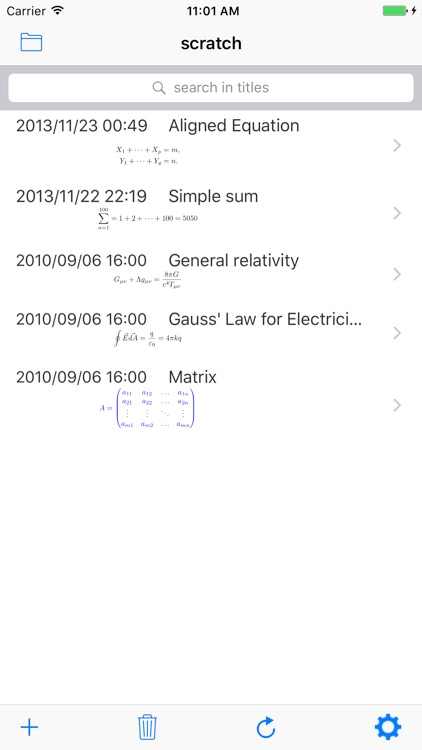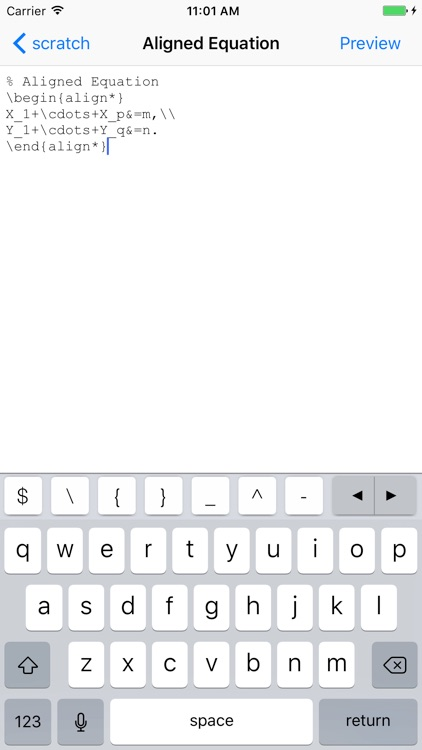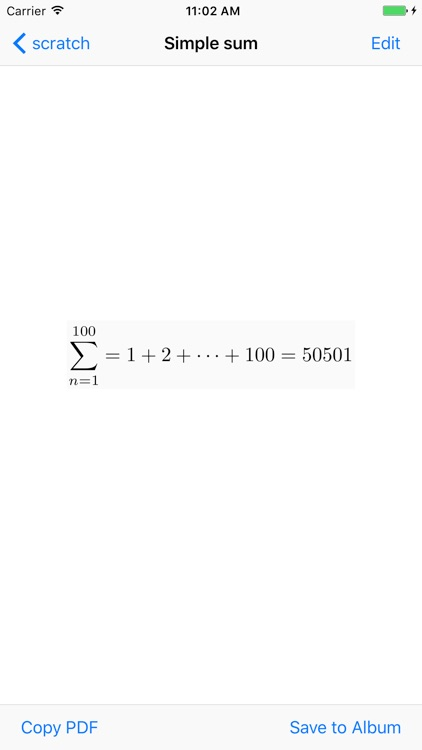TeX Equation typesets math equations/formulas with built-in TeX engine. It is a great companion to applications like Pages/Keynote.### App Details

Version
17.09
Rating
(10)
Size
43Mb
Genre
Last updated
September 25, 2017
Release date
September 21, 2010

### App Store Description

TeX Equation typesets math equations/formulas with built-in TeX engine. It is a great companion to applications like Pages/Keynote.

- Customized keyboard includes frequently used keys
- Support LaTeX / AMS LaTeX macros
- Support PDF output
- Real time equation preview
- Copy equation image
- Export equation image to album
- Search in equation list
- Customize foreground color for equations (background is always transparent).
- User can define their own macros and code snippets for reuse
- User can group equations into different lists

Recommendations:
- Acquaintance with TeX